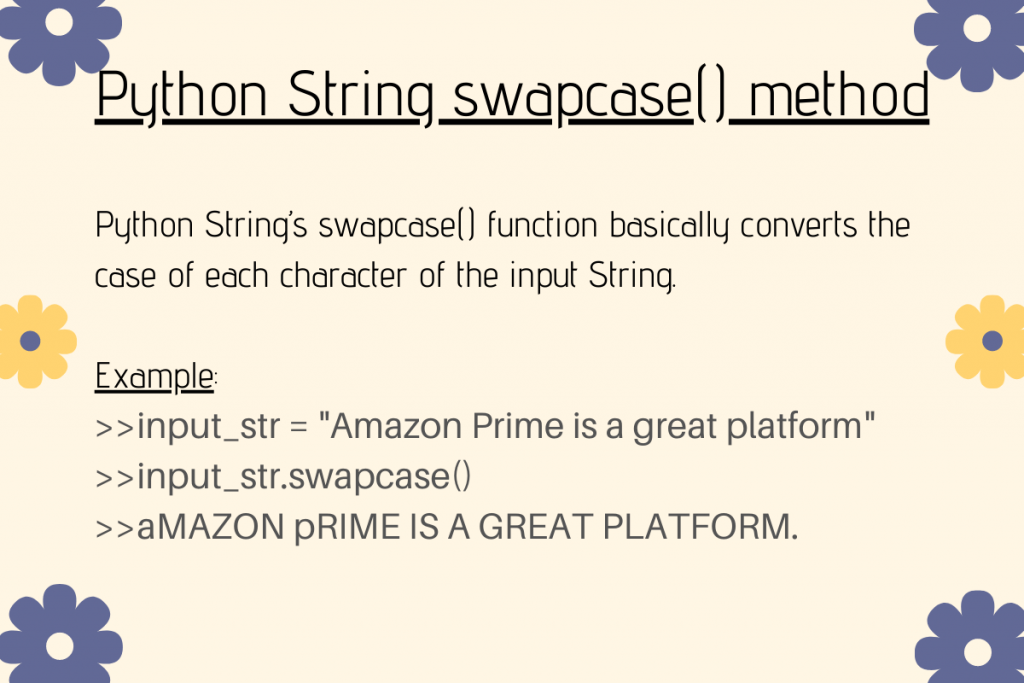# Python String swapcase() methodIn this article, we will unveil the Python String swapcase() function in detail. Python String provides us with a number of built-in methods to manipulate the input data for further operations. Let’s get started with understanding this method.

## Getting started with Python String swapcase() method

Python String `swapcase()` function converts the case of each character of the input String. It converts all the lowercase characters to uppercase characters and vice-versa.

Syntax:

```input_string.swapcase()
```

Example 1:

```input_str = "Amazon Prime is a great platform."
res=input_str.swapcase()
print(res)
```

Output:

```aMAZON pRIME IS A GREAT PLATFORM.
```

Example 2:

```input_str = "AMAZON PRIME IS A GREAT PLATFORM!!"
res=input_str.swapcase()
print(res)
```

Output:

```amazon prime is a great platform!!
```

## NumPy String swapcase() function

Python’s NumPy module provides us with a function to convert the case of letters of the input.

The `numpy.char.swapcase()` function converts the case of the characters of the input data in an element-wise fashion.

Syntax:

```numpy.char.swapcase(input_array)
```

Example:

```import numpy

inp_arr = numpy.array(['Engineering', 'Science', 'Commerce', 'A5Z'])

print ("Elements of Input array:\n", inp_arr)

res = numpy.char.swapcase(inp_arr)
print ("Elements of array after swapping the case of each one:\n", res)
```

Note: Python `numpy.array()` function creates an array of the input elements.

Output:

```Elements of Input array:
['Engineering' 'Science' 'Commerce' 'A5Z']
Elements of array after swapping the case of each one:
['eNGINEERING' 'sCIENCE' 'cOMMERCE' 'a5z']
```

## Pandas Series swapcase() function

Python Pandas module contains various kinds of data structures to represent the data. One such data structure being Series.

Pandas’s Series `swapcase()` function enables us to change/convert the case of every string present in the Series. As compared to Python string swapcase() function, Pandas swapcase() can work with files too as demonstrated in the example below.

Syntax:

```<Series>.str.swapcase()
```

Input .csv file:

Example:

```import pandas

inp_file["Name"] = inp_file["Name"].str.swapcase()

print(inp_file)
```

The `pandas.read_csv(file_path)` method is used to input and read a file with .csv extension.

inp_file[“Name”].str.swapcase() method converts the case of every string present under the column Name of the .csv file.

Output: